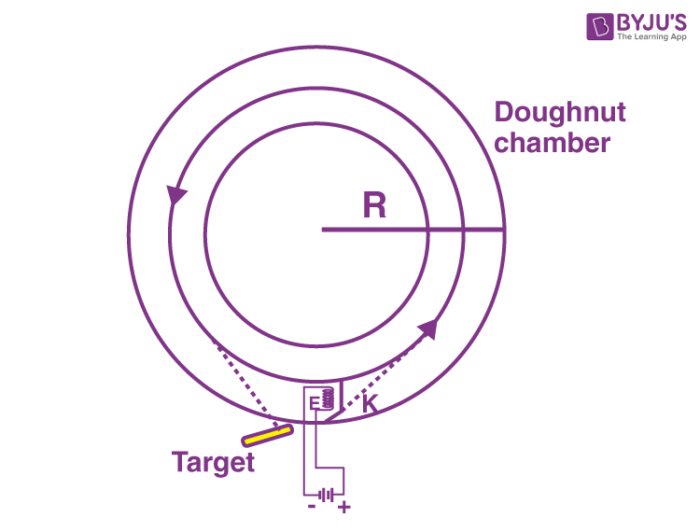A machine that uses electromagnetic fields to drive high-speed, high-energy charged particles in well-defined beams is known as a particle accelerator. In this article, let us know about one such particle accelerator known as Betatron.

## What is Betatron?

Betatron is a type of particle accelerator and is used to accelerate electrons. It uses the electric field induced by a varying magnetic field to accelerate electrons to high speed in a circular orbit. The word betatron derives from the fact that high-energy electrons are often called β-particles. The betatron was the first important machine for producing high-energy electrons.

It was first developed in 1935 by Max Steenbeck in Germany to accelerate electrons, but the concepts ultimately originate from Rolf Widerøe, whose development of an induction accelerator failed due to the lack of transverse focusing. In 1940, the successful betatron was developed at the University of Illinois by the American physicist Donald W. Kerst. A simple representation of the betatron is shown in the figure below.### Betatron Oscillation

Betatron oscillation refers to the oscillations of particles about their stable or equilibrium orbits in all circular accelerators. These are the stable oscillations about the equilibrium orbit in the horizontal and vertical planes.

Hill’s Equation describes this type of traverse motion by:

$$\begin{array}{l}\large \frac{d^{2}x}{ds^{2}} + K (s) x = 0\end{array}$$

### Applications of Betatron

Some applications are stated below:

• Provides high energy beam electrons of about 300 MeV.
• Used as a source of X-rays and gamma rays if the electron beam is made to direct on a metal plate.
• The X-rays produced with the help of betatron can be used in industrial and medical fields.
• High energy electrons can be used in particle physics.
• Possible solar flare mechanism.

### Limitations of Betatron

This type of particle has maximum energy, and can be limited by the strength of the magnetic field due to the saturation of iron and by the practical size of the magnet core.

It is actually a transformer, which acts as the secondary coil of the transformer. This accelerates the electrons in the vacuum tube around a circular path. It works under constant electric fields and variable magnetic fields.

## Frequently Asked Questions – FAQs

Q1

### What is a particle accelerator?

It is a machine that uses electromagnetic fields to drive high-speed, high-energy charged particles in well-defined beams.
Q2

### What are the types of particle accelerators?

The following are the types of particle accelerators:

• Electrostatic particle accelerator
• Electrodynamics particle accelerator
Q3

### What are the types of electrodynamics or electromagnetic particle accelerator?

Following are the types of electrodynamics or electromagnetic particle accelerator:

• Linear accelerator
• Circular accelerator
Q4

### Betatron is an example for which type of accelerator?

Betatron is an example of an electrodynamics or electromagnetic particle accelerator.
Q5

### State true or false: Betatron can provide high energy beam electrons of about 300 MeV.

True.
Hope you have understood about betatron and its applications. Stay tuned with BYJU’S to know about various interesting topics!.